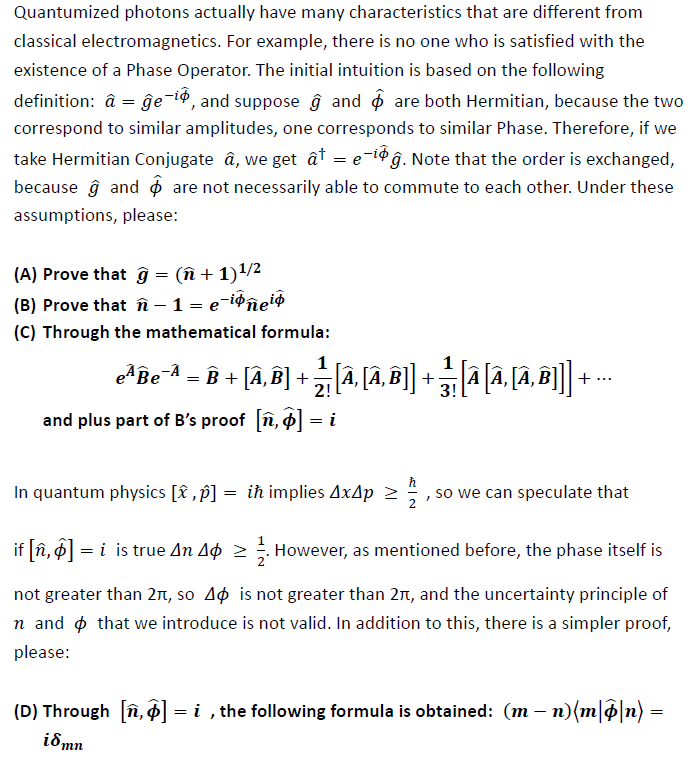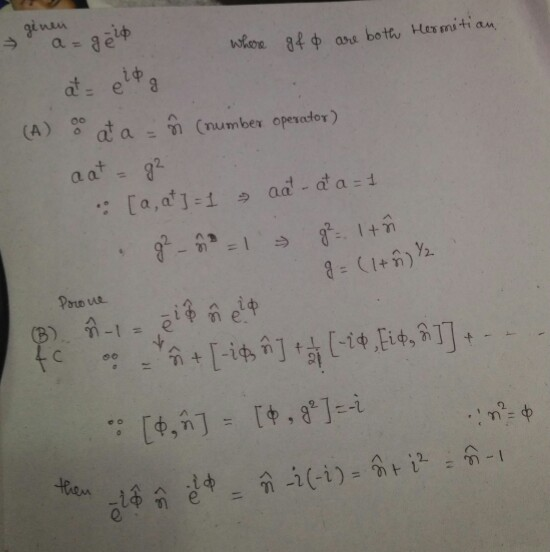Homework Help Question & Answers

# Quantumized photons actually have many characteristics that are different from classical electrom...Quantumized photons actually have many characteristics that are different from classical electromagnetics. For example, there is no one who is satisfied with the existence of a Phase Operator. The initial intuition is based on the following definition: а-де-іф, and suppose and ф are both Hermitian, because the two correspond to similar amplitudes, one corresponds to similar Phase. Therefore, iT we take Hermitian Conjugate a, we get at-e-ing Note that the order is exchanged, because g and ф are not necessarily able to commute to each other. Under these assumptions, please: 1/2 (A) Prove that g - (n + 1) (B) Prove that ñ-1-e-14hel (C) Through the mathematical formula: and plus part of B's proof n,##### Add Answer of: Quantumized photons actually have many characteristics that are different from classical electrom...
More Homework Help Questions Additional questions in this topic.

• #### Identify the domain and range of the following: f(x) = 4-x^2, -5<x<-1 3/2x + 3/2, -1<x<3 (both "<" signs are actually less than or equal to) x+3, 3<x<10 (x<10 is actually less than or equal to) All three are in a se

Need Online Homework Help?

Get FREE EXPERT Answers
WITHIN MINUTES
Related Questions